Published: 15 August 2015

Xuemei Huang1
Lei’an Zhang2
Guangming Yuan3
Na Wang4
1, 2, 3, 4College of Mechanical Engineering, Shandong University of Technology, Shandong, China
Corresponding Author:
Xuemei Huang
Views 149

#### Abstract

A new dual-excitation fatigue loading system of wind turbine blades was designed in this paper. However, the two excitations and blade constituted a complicated non-liner energy transferring system in which the vibration coupling effect would influence the sequent accurate control of fatigue test. To study the mechanism of the coupling system mentioned above, the electromechanical coupling mathematical model was established by simplifying the loading system rationally and the factors affecting the vibration coupling were obtained accordingly. Then the simulation model of the system was built in Matlab/Simulink environment to mainly analyze the basic influence laws of the motor speed and the initial phase difference of two excitations. Finally, a small dual-excitation fatigue loading system was established to verify the correctness of the mathematical and simulation model. It could be concluded that the results of on-site test were consistent with the results of simulation.

## 1. Introduction

As an important renewable energy technology, wind power generation has many ecological and economic benefits. According to the report Technology Roadmaps – Wind energy released by International Energy Agency, by the mid-21st century, the proportion of wind power generation in global power supply will rise from the present 2 % to 12 % . Ina wind turbine generator, blades are the main part bearing the wind power. A large number of accidents and research results show that due to the impact of alternating load, fatigue is one of the main failure mechanisms for blades [2-3]. Thus, fatigue test of a wind turbine blade is an effective and reliable method to detect weaknesses in a blade’s design .

Fatigue tests on blades are typically conducted by imposing an excitation mounted at 70 % of the blade length along the span wise direction and making the blade and excitation resonate together . However, this test method has deficiencies of long test cycle and lacking of driving capability etc. In this paper, a dual-excitation fatigue loading system of wind turbine blades was developed to solve the problems mentioned above. However, the two excitations and blade constitute a complicated non-liner energy transferring system, in which the vibration coupling effect will influence the distribution of flexural moment and resonance of whole system and further more lead to inaccurate control of fatigue test. So the coupling mechanism of dual-excitation fatigue loading system need to be investigated.

For dual-excitation vibration system, Blekhan first proposed the synchronous control theory of dual-excitation vibration machine . After that, the coupling characteristics of dual-exciter vibration mechanical system have been investigated by many researchers [7-10]. However, from the current literatures, it could be concluded that in the field of fatigue loading tests for wind turbine blades, studies on the vibration coupling mechanism of two exciters have not been carried out both in domestic and abroad.

### 2.1. Establishing the mathematical model the mechanical vibration system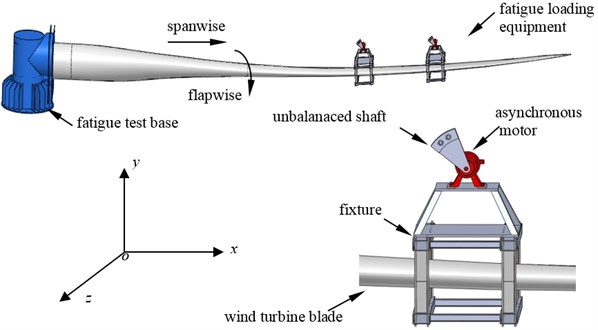The dynamic model of the dual-excitation fatigue loading system is shown in Fig. 2. In order to simplify the system, it was assumed that the damping force suffered by the blade in each direction was a linear function of the velocity, and the elastic force was also a linear function of the displacement.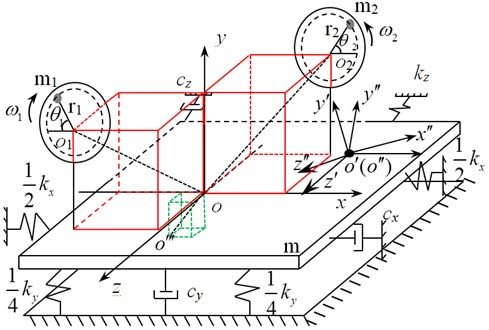In Fig. 2, $oxyz$ is the absolute coordinate system, $o\text{'}x\text{'}y\text{'}z\text{'}$ and $o\text{'}\text{'}x\text{'}\text{'}y\text{'}\text{'}z\text{'}\text{'}$ are the moving coordinate system, $o\text{'}\text{'}\text{'}$ is the centroid of the whole vibration system (include the blade and the two set of loading equipment), $m$ is the total weight of the blade and two sets offatigue loading equipment. ${o}_{i}$ is the rotary center of the unbalanced shaft $i$, ${m}_{i}$ is the weight of the unbalanced shaft $i$, ${r}_{i}$ is the equivalent arm length of the unbalanced shaft $i$, ${\theta }_{i}$ and ${\omega }_{i}$ are the rotary angle and angular velocity of the the unbalanced shaft $i$ and ${\omega }_{i}=\stackrel{˙}{{\theta }_{i}}$ ($i=$ 1, 2).

$x$, $y$, $z$, ${\phi }_{i}$ and ${\theta }_{i}$ ($i=$ 1, 2)were regarded as generalized coordinates, then according to Lagrange equation, the dynamic equation of the dual-excitation fatigue loading system is established as follows:

1
$\left\{\begin{array}{l}\left(m+{\sum }_{i=1}^{2}{m}_{i}\right)\stackrel{¨}{x}+{k}_{x}x+{c}_{x}\stackrel{˙}{x}=0,\\ \left(m+{\sum }_{i=1}^{2}{m}_{i}\right)\stackrel{¨}{y}+{\sum }_{i=1}^{2}{m}_{i}{r}_{i}\left({\stackrel{¨}{\theta }}_{i}\mathrm{cos}{\theta }_{i}-{\stackrel{˙}{\theta }}_{i}^{2}\mathrm{sin}{\theta }_{i}\right)+{k}_{y}y+{c}_{y}\stackrel{˙}{y}=0,\\ \left(m+{\sum }_{i=1}^{2}{m}_{i}\right)\stackrel{¨}{z}+{k}_{z}z+{c}_{z}\stackrel{˙}{z}=0,\\ \left(m{l}_{01}^{2}+{\sum }_{i=1}^{2}{m}_{i}{l}_{i1}^{2}+{J}_{01}\right){\stackrel{¨}{\phi }}_{1}+\left(m{l}_{01}{l}_{02}\mathit{sin}{\beta }_{01}\mathrm{sin}{\beta }_{02}\\ -{\sum }_{i=1}^{2}{m}_{i}{l}_{i1}{l}_{i2}\mathrm{cos}{\beta }_{i1}\mathrm{cos}{\beta }_{i2}\right){\stackrel{¨}{\phi }}_{2}+{k}_{{\phi }_{1}}{\phi }_{1}+{c}_{{\phi }_{1}}{\stackrel{˙}{\phi }}_{1}\\ ={\sum }_{i=1}^{2}{m}_{i}{r}_{i}{l}_{i1}\mathrm{cos}{\beta }_{i1}\left({\stackrel{¨}{\theta }}_{i}\mathrm{cos}{\theta }_{i}-{\stackrel{˙}{\theta }}_{i}^{2}\mathrm{sin}{\theta }_{i}\right),\\ \left(m{l}_{02}^{2}+{\sum }_{i=1}^{2}{m}_{i}{l}_{i2}^{2}+{J}_{02}\right){\stackrel{¨}{\phi }}_{2}+\left(m{l}_{01}{l}_{02}\mathrm{sin}{\beta }_{01}\mathrm{sin}{\beta }_{02}\\ -{\sum }_{i=1}^{2}{m}_{i}{l}_{i1}{l}_{i2}\mathrm{cos}{\beta }_{i1}\mathrm{cos}{\beta }_{i2}\right){\stackrel{¨}{\phi }}_{1}+{k}_{{\phi }_{2}}{\phi }_{2}+{c}_{{\phi }_{2}}{\stackrel{˙}{\phi }}_{2}\\ ={\sum }_{i=1}^{2}{m}_{i}{{r}_{i}l}_{i2}\mathrm{cos}{\beta }_{i2}\left({\stackrel{¨}{\theta }}_{i}\mathrm{cos}{\theta }_{i}-{\stackrel{˙}{\theta }}_{i}^{2}\mathrm{sin}{\theta }_{i}\right),\\ {J}_{i}{\stackrel{¨}{\theta }}_{i}+{m}_{i}{r}_{i}\mathrm{cos}{\theta }_{i}\left(\stackrel{¨}{y}+{\stackrel{¨}{\phi }}_{1}{l}_{i1}\mathrm{cos}{\beta }_{i1}-{\stackrel{¨}{\phi }}_{2}{l}_{i2}\mathrm{cos}{\beta }_{i2}+{\stackrel{¨}{\theta }}_{i}{r}_{i}\mathrm{cos}{\theta }_{i}-{\stackrel{˙}{\theta }}_{i}^{2}{r}_{i}\mathrm{sin}{\theta }_{i}\right)\\ ={T}_{{m}_{i}},\left(i=1,2\right),\end{array}\right\$

where, ${\phi }_{i}$ is the included angle of $oxyz$ and two moving coordinate system, respectively, ${l}_{i1}$ is the projection of $o{o}_{i}$ in the plane $yoz$ and ${l}_{i2}$ is the projection of $o{o}_{i}$ in the plane $xoy$. ${\beta }_{i1}$ is the include dangle of ${l}_{i1}$ and the positive direction of $z$-axis, ${\beta }_{i2}$ is the included angle of ${l}_{i2}$ and the positive direction of $x$-axis and ${\beta }_{0i}$ is the include dangle of ${l}_{0i}$ and the negative direction of $y$-axis, ${k}_{x}$,…, ${k}_{y}$, ${k}_{z}$ and ${k}_{\phi i}$ are the stiffness coefficient of $x$, $y$, $z$ and ${\phi }_{i}$ direction, respectively, ${c}_{x}$, ${c}_{y}$, ${c}_{z}$ and ${c}_{\phi i}$ are the damping coefficient of $x$, $y$, $z$ and ${\phi }_{i}$ direction, respectively, ${J}_{oi}$ is the rotational inertia of the system around $x$ axis and $z$ axis, ${J}_{i}$ is the rotational inertia of the asynchronous motor $i$, ${T}_{mi}$ is the electromagnetic torque of the shaft of the asynchronous motor $i$ ($i=$ 1, 2 for all the parameters above). ${l}_{01}$ and ${l}_{02}$ are the projection of $oo\text{'}\text{'}\text{'}$ in the plane $yoz$ and plane $xoy$, respectively.

### 2.2. The mathematical model of three-phase asynchronous motor

In the fatigue loading equipment, each unbalanced shaft was driven by a three-phase asynchronous motor, which would generate the loading force. So the mathematical model of the three-phase asynchronous-motor was also needed to be built. However, the model of asynchronous motor in the case of three-phase was complicated, so in practice, the mathematical model based on two-phase synchronous rotating coordinate system $\alpha \beta$ was applied to represent the original model in $abc$ system .

The coordinate transformation equation of three-phase asynchronous motor from $abc$ system to $\alpha \beta$ system is expressed by:

2
$\left\{\begin{array}{l}{i}_{\alpha }=\sqrt{\frac{2}{3}}{i}_{a}-\frac{1}{2}\left({i}_{b}+{i}_{c}\right),\\ {i}_{\beta }=\frac{\sqrt{3}}{2}\left({i}_{b}-{i}_{c}\right).\end{array}\right\$

The voltage equation of three-phase asynchronous motor based on $\alpha \beta$ system is expressed by:

3
$\left\{\begin{array}{l}{u}_{\alpha 1}={r}_{1}{i}_{\alpha 1}+p{\mathrm{\Psi }}_{\alpha 1},\\ {u}_{\beta 1}={r}_{1}{i}_{\beta 1}+p{\mathrm{\Psi }}_{\beta 1},\\ {u}_{\alpha 2}={r}_{2}{i}_{\alpha 2}+p{\mathrm{\Psi }}_{\alpha 2}+{\mathrm{\Psi }}_{\beta 2}{\omega }_{r},\\ {u}_{\beta 2}={r}_{2}{i}_{\beta 2}+p{\mathrm{\Psi }}_{\beta 2}+{\mathrm{\Psi }}_{\alpha 2}{\omega }_{r}.\end{array}\right\$

The flux linkage equation of three-phase asynchronous motor based on $\alpha \beta$ system is expressed by:

4
$\left\{\begin{array}{l}{\mathrm{\Psi }}_{\alpha 1}={L}_{s}{i}_{\alpha 1}+{L}_{m}{i}_{\alpha 2},\\ {\mathrm{\Psi }}_{\beta 1}={L}_{s}{i}_{\beta 1}+{L}_{m}{i}_{\beta 2},\\ {\mathrm{\Psi }}_{\alpha 2}={L}_{r}{i}_{\alpha 2}+{L}_{m}{i}_{\alpha 1},\\ {\mathrm{\Psi }}_{\beta 2}={L}_{r}{i}_{\beta 2}+{L}_{m}{i}_{\beta 1}.\end{array}\right\$

The electromagnetic torque and the load torque equation is expressed by:

5
${T}_{e}={n}_{p}{L}_{m}\left({i}_{\beta 1}{i}_{\alpha 2}-{i}_{\beta 2}{i}_{\alpha 1}\right),$
6
${T}_{e}={T}_{L}+\frac{J}{{n}_{p}}\bullet \frac{d{\omega }_{r}}{dt},$

where, ${r}_{1}$ and ${r}_{2}$ are the winding resistance of the stator and the rotor, ${i}_{\alpha 1}$ and ${i}_{\beta 1}$ are the terminal currents of the stator in $\alpha \beta$ system, ${i}_{\alpha 2}$ and ${i}_{\beta 2}$ are the terminal currents of the rotor in $\alpha \beta$ system, ${u}_{\alpha 1}$ and ${u}_{\beta 1}$ are the terminal voltages of the stator in $\alpha \beta$ system, ${u}_{\alpha 2}$ and ${u}_{\beta 2}$ are the terminal voltages of the rotor in $\alpha \beta$ system. ${\omega }_{r}$ is the angular velocity of the rotor, ${\mathrm{\Psi }}_{\alpha 1}$ and ${\mathrm{\Psi }}_{\beta 1}$ arethe flux linkage of the stator in $\alpha \beta$ system, ${\mathrm{\Psi }}_{\alpha 2}$ and ${\mathrm{\Psi }}_{\beta 2}$ arethe flux linkageof the rotorin $\alpha \beta$ system, ${L}_{s}$ and ${L}_{r}$ are the self-inductance of the stator and rotor winding resistance, ${L}_{m}$ is the mutual-inductance of the stator and rotor winding, ${T}_{e}$ is the electromagnetic torque, ${T}_{L}$ is the load torque, ${n}_{p}$ is the number of pole pairs, $p$ is the differential operator.

Eqs. (1)-(6) constituted the electromechanical coupling mathematical model of the dual-excitation fatigue loading system of wind turbine blades. This mathematical model was a non-ideal space coupling model and described the space coupling effect between the power system and vibration system during the loading process. It could be seen that the rotary driving speeds of motors, the initial phase difference of two unbalanced shafts and the mechanical properties of asynchronous motors, etc. were the factors affecting the vibration coupling.

## 3. Simulation analysis of the vibration coupling process

For the limited space, the influence law of driving speeds of motors and the initial phase difference of two unbalanced shafts were analyzed in simulation software Matlab/Simulink. Considering the sequent on-site test condition, the parameters of a small wind turbine blade were adopted for simulation. Moreover, symmetric installation was applied for the two set of loading equipment and the symmetric center was the point located at 70 % of the blade length along the span wise direction, namely ${l}_{12}$ was equal to ${l}_{22}$ in Eq. (1).

The parameters of simulation were set: $m=$ 29 kg, ${m}_{1}={m}_{2}=$2 kg, $r=$ 0.15 m, ${l}_{01}=$ 0.05 m, ${l}_{02}=$0.25 m, ${l}_{11}={l}_{21}=$0.13 m, ${l}_{12}={l}_{22}=$ 0.32 m, ${\beta }_{01}=$45°, ${\beta }_{02}=$2.5°, ${\beta }_{11}=$ 42°, ${\beta }_{12}=$160°, ${\beta }_{21}=$138°, ${\beta }_{22}=$ 20°.

The initial phase difference of two unbalanced shafts was set to $\pi /$2. The rotary driving speeds of two asynchronous motors were the same and set to 150 r/min, 180 r/min, 210 r/min, 230 r/min and 260 r/min, respectively. The simulation result is shown in Fig. 3.

Fig. 3Phase difference change curve sat different rotary driving speed of the motors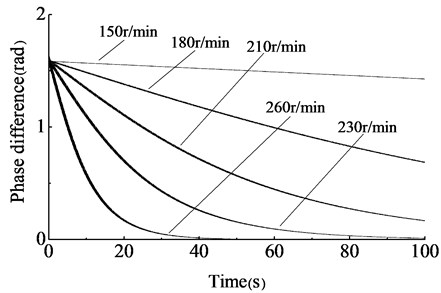In Fig. 3, under different rotary driving speeds, phase differences of two unbalanced shafts all gradually converged to zero. When the driving speeds of motors were 260 r/min, the phase difference converged to zero with the shortest time, about 40 s. As speeds decrease, more time would be needed for phase difference converging to zero. When the rotary driving speeds were 150 r/min, the phase difference converged to zero with long time, over 100 s. Furthermore, when the rotary driving speeds were 260 r/min, with the phase difference gradually converging to zero, the amplitude in $y$ direction become stabilizing after a period of fluctuation, and the simulation result is shown in Fig. 4.

Fig. 4The amplitude in y direction, the rotary driving speed = 260 r/min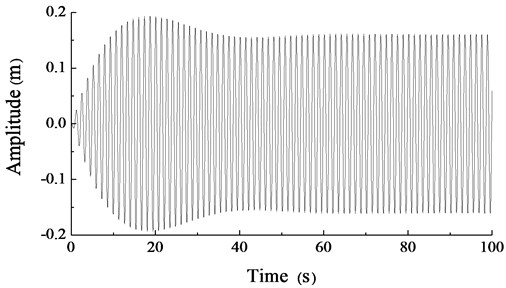When the rotary driving speeds of motors were set to 230 r/min and the initial phase difference of two unbalanced shafts was set to –5$\pi /$6, $\pi /$2, –$\pi /$6, $\pi /$6, $\pi /$2, 5$\pi /$6 and $\pi$, respectively. The simulation result is shown in Fig. 5.

In Fig. 5, the phase differences of the two unbalanced shafts gradually converged to zero after a period of time even the initial values were a bit large. However, when the initial value was $\pi$, the phase difference would converge to zero with longer time compared to others, and the vibration system is difficult to achieve the synchronization status. To obtain more stable amplitude, it should be avoid that the initial phase difference of the two unbalanced shafts was $\pi$.

When the rotary driving speeds of the two asynchronous motors were different from each other and set to 230 r/min and 217 r/min, respectively, the simulation result of the phase difference is shown in Fig. 6, and the amplitude result in y direction is shown in Fig. 7.

Fig. 5Phase difference change curves under different initial values, the rotary driving speed = 230 r/min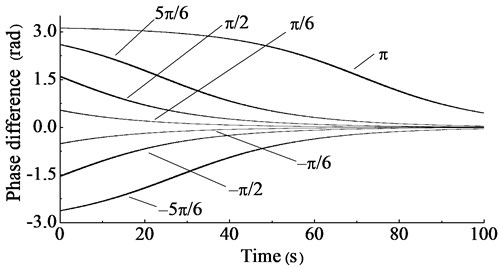Fig. 6The phase difference simulation result of two unbalanced shafts driven by different speeds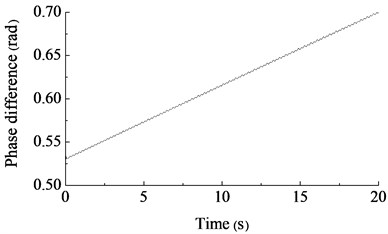Fig. 7The amplitude simulation curve in y direction under the different speed of two motors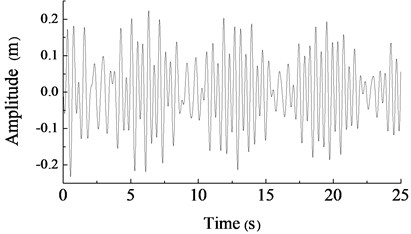In Fig. 6 and Fig. 7, if the initial rotary driving speeds of two unbalance shafts were high and their speed difference was large, the two set of fatigue loading equipment could not achieve synchronization status and the amplitude in y direction could not stabilize. To obtain stable amplitude, the rotary driving speeds of two asynchronous motors should be as similar as possible, so the synchronized control of two loading sources was indispensable.

## 4. Test verification

To verify the correctness of the theoretical analysis and the simulation results, a small-scale dual-exaction fatigue loading system of a wind turbine blade was established. The on-site test is shown in Fig. 8 and part of test parameters is shown in Table 1.

Table 1Test parameters

 Parameter Value (or type) Length of the blade 2.3 m Weight of the blade (kg) 25 Weight of the unbalanced shaft (kg) 2 Equivalent arm length of the unbalanced shaft (m) 0.15 Power of the motor (kw) 0.75 Temperature (°C) 15 Humidity (RH) 40 %

The rotary driving speeds and phases of two unbalanced shafts were measured real-time by gear speed sensors (type: CORON-CTS). Gears with 120 teeth were installed on the motor shaft so that the gears and the motor shaft can rotate synchronously. During the measuring, each gear speed sensor produced a pulse when a tooth of gear was detected, so the driving speeds and phases of the unbalanced shafts could be obtained. A proximity switch was installed below every unbalanced shaft to realize the reset of phase. The amplitude in $y$ direction was measured by a laser range meter (type: ADSL-30) and the amplitude data was transferred real-time to the controller.

In this paper, Siemens S7-200 PLC was applied as the controller which calculated and analyzed the received gear pulses and amplitude data, then transmitted them to the host computer via RS485 bus. The interface of host computer was designed by LabVIEW.

During the test process, the rotary driving speeds of the two asynchronous motors were both set to 230 r/min, and the initial phase difference of the two unbalanced shafts was set to $\pi /$6. For other parameters, see the set of parameters in simulation section. The test results are shown in Fig. 9 and Fig. 10.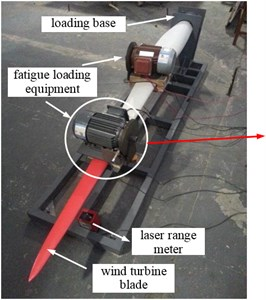a) The whole test scene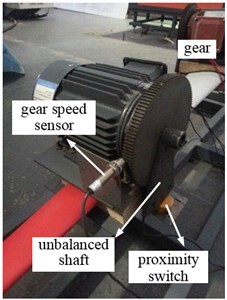b) Partial enlarged

Fig. 9Test curve of phase difference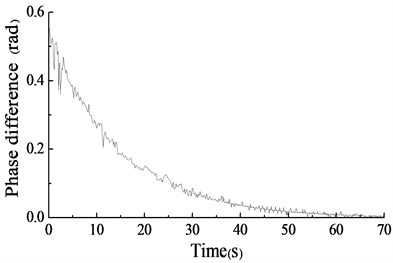Fig. 10The amplitude test curve in y direction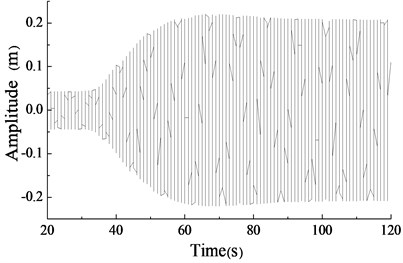In Fig. 9 and Fig. 10, the phase difference of two unbalanced shafts gradually converged from $\pi /$6 to zero. And the amplitude in y direction gradually tended to be stable and finally kept between ±0.25 m.

When the rotary driving speeds of two asynchronous motors were different and set to 230 r/min and 217 r/min, respectively, the test result are shown in Fig. 11 and Fig. 12.

The test result showed that the phase difference of two unbalanced shafts gradually increased if their initial driving speed difference was large. And the amplitude of the blade in $y$ direction was disordered. The two set of fatigue loading equipment were difficult to achieve the synchronization status automatically. The test results above were consistent with the simulation results basically.

Fig. 11The phase difference test result of two unbalanced shafts driven by different speeds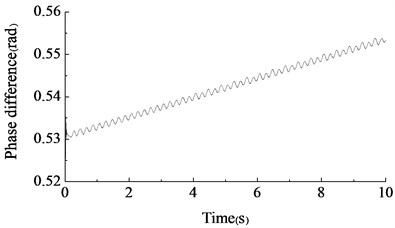Fig. 12The amplitude test curve in y direction under the different speed of two motors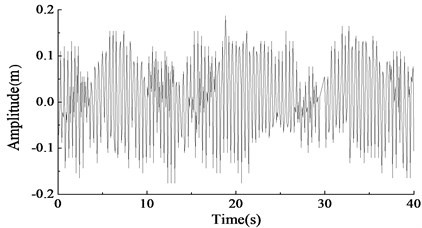## 5. Conclusion

The vibration characteristics of dual-excitation fatigue loading system of wind turbine blades was investigated, conclusions can be drawn as follows:

1) By establishing the electromechanical coupling mathematical model of the dual-excitation fatigue loading system, it was found that the main factors influencing the coupling characteristics included the initial phase difference, rotary driving speed and eccentric moment of two unbalanced shafts, the location of two set of fatigue loading equipment and the mechanical properties of asynchronous motor etc.

2) By establishing the simulation model in simulation software Matlab/Simulink, the phase differences of the two unbalanced shafts and the amplitude of the blade in $y$ direction was obtained visually.When the rotary driving speeds of two asynchronous motors were the same, the phase difference of two unbalanced shafts gradually converged to zero, even the initial phase difference of them was not zero. What more, the higher the driving speed of motor was, the faster the phase difference converged to zero. Finally, the vibration system could step into synchronous status and at the vibrating direction, the stable amplitude could be obtained. However, it should be avoid that the initial phase difference of the two unbalanced shafts was $\pi$ because a long period of time would be needed to reach the vibration synchronous status.When the initial rotary driving speeds of two asynchronous motors were high and their speed difference was large, the two set of fatigue loading equipment could not achieve synchronization status and the amplitude in $y$ direction was disordered.

3) The results of the on-site test were consistent with the results of the simulation, which verified the correctness of the mathematical model built in this paper.

25 March 2015
Accepted
15 July 2015
Published
15 August 2015
SUBJECTS
Flow induced structural vibrations
Keywords